#### You may also like### Prompt Cards

These two group activities use mathematical reasoning - one is numerical, one geometric.### Consecutive Numbers

An investigation involving adding and subtracting sets of consecutive numbers. Lots to find out, lots to explore.### Exploring Wild & Wonderful Number Patterns

EWWNP means Exploring Wild and Wonderful Number Patterns Created by Yourself! Investigate what happens if we create number patterns using some simple rules.

# Dice and Spinner Numbers

##### Age 7 to 11 Challenge Level: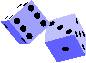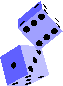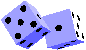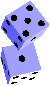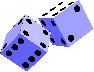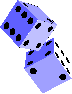We often roll dice for games and sometimes we're lucky enough to use two dice at a time and add them together to get a much higher number.
I happen to have a dice in front of me at this moment and it's showing a 3. If I had another dice I might get a 1, 2, 3, 4, 5 or 6 on that one and so the total would be 4, 5, 6, 7, 8 or 9.

Let's play around with this idea for a while. I think that it'd be good to write some things down. So we might have something like:-

3 + 1 = 4
3 + 2 = 5
3 + 3 = 6
3 + 4 = 7
3 + 5 = 8
3 + 6 = 9

all that seem fine. But of course the first dice might have been a five in which case we would have:-

5 + 1 = 6
5 + 2 = 7
5 + 3 = 8
5 + 4 = 9
5 + 5 = 10
5 + 6 = 11

We could of course have a look at all the different possibilities whether the 1st. is a 1, 2, 3, 4, 5 or 6.
So we would have 6 tables like those above giving 36 additions altogether.

Some of the answers occur several times, for example the answer 6 would come from:-

1 + 5
2 + 4
3 + 3
4 + 2
5 + 1

The top two look a bit like the last two and since the two dice might be the same colour let's just take those first three results.

The 1 + 5, 2 + 4, 3 + 3 could also be changed so that instead of adding these dice numbers together we'll multiply them!

1 x 5 = 5
2 x 4 = 8
3 x 3 = 9

This is quite nice let's take it a bit further and not just have two dice but as many as we like!!!

BUT suppose that we wanted the total when we added them still to be 6 like in our first example.

We could have:-
2 + 2 + 2
1 + 1 + 1 + 1 + 2
3 + 1 + 1 + 1

and so on. Have a go and see what numbers you could come up with that would give a total of 6 by adding no matter how many dice you had.

If you could then put these numbers together and multiply them this time we'd have a new set of answers. My examples would give :-

 2 + 2 + 2 2 x 2 x 2 = 8 1 + 1 + 1 + 1 + 2 1 x 1 x 1 x 1 x 2 = 2 3 + 1 + 1 + 1 3 x 1 x 1 x 1 = 3

It might be good to gather together the answers and put them in an order.

But wait a minute we have not got many answers because we can only use 2, 3, 4, 5 or 6 dice. But we could just have one dice that rolls a 6. We would not have to add anything to this, and so we would not have to multiply this by anything and the final answer would just be a six!!

All this has come from getting a total of 6 by addition when rolling ordinary dice.

You might like to check that you've got all the possibilities and then get just the final answers together. All coming from multiplying together numbers which would ADD up to 6.

Some games use a spinner instead of a dice and this can have a different number of sides, a hexagon one would obviously be like using an ordinary dice. But suppose we had a seven-sided and an eight-sided available. Then when we ask the question about totals that made 8 we could do so much more.
Let's have a look at the kinds of numbers we would be having:-

1 + 7 = 8 and 1 x 7 = 7
2 + 2 + 2 + 2 = 8 and 2 x 2 x 2 x 2 = 16
3 + 1 + 3 + 1 = 8 and 3 x 1 x 3 x 1 = 9
and 8 on its own!

See if you can find all the ones that could be used this time and what happens when we multiply them like the 4 I've started with.

When you have them all then arrange them and start exploring. There's other totals when adding you could aim for, it would be a good idea not to go above 12 as the total when adding as I guess there are so very many ways with lots of spinners of different sizes that you could use.

Now you just need to be reminded to ask yourself "I wonder what would happen if I ........?"